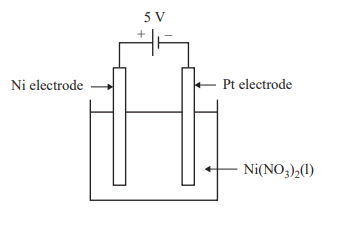Electrolysis (2021 VCE ) 1) An electrolysis cell consumed a charge of 4.00 C in 5.00 minutes. This represents a consumption of A. 4.15 × 10−5 mol of electrons. B. 2.07 × 10−4 mol of electrons. C. 1.93 × 104 mol of electrons. D. 2.41 × 104 mol of electrons. Solution2) The electrolysis of water is used to produce oxygen, O2, gas. The O2 gas produced is piped into a 200.0 L fixed-volume gas storage container at 22.0 °C. When more O2 is added, the pressure in the container increases. What mass of O2 needs to be added to increase the pressure by 250.0 kPa? A. 1.53 × 10−3 g B. 6.37 × 10−1 g C. 6.53 × 102 g D. 8.75 × 103 g Solution3) An electrolysis cell with a 5 V power supply is shown below.1 F is equivalent to the charge on 1 mol of electrons. The mass of nickel, Ni, that can be electroplated onto the platinum, Pt, electrode with 320 F of charge is A. 9.73 × 10−2 g B. 1.95 × 10−1 g C. 9.39 × 103 g D. 1.88 × 104 g Solution4) Using the electrochemical series, which one of the following changes to the electrolysis cell, shown in 3) above, may reduce the amount of Ni electroplated onto the Pt electrode? A. replacing the Ni electrode with a Cu electrode B. replacing Ni(NO3)2(l) with 1 M Ni(NO3)2(aq) C. replacing the Pt electrode with Pb(s) D. replacing Ni(NO3)2(l) with NiCl2(l) Solution